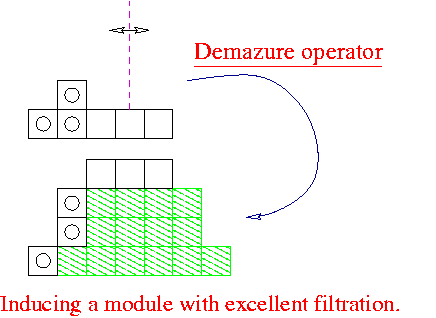# Wilberd van der Kallen

## Geometry and Quantum Theory clusterMathematisch Instituut
Universiteit Utrecht
P.O.Box 80.010
3508 TA UTRECHT
The Netherlands

### Location:

"Hans Freudenthal" building.

### E-mail:

W.vanderKallen@uu.nl

## Two notes

Wilberd van der Kallen,
Computing some KL-polynomials for the poset of B×B-orbits in group compactifications (July 2002)
Wilberd van der Kallen,
Complexity of an extended lattice reduction algorithm (1997-1998)

## Programs

### Hecke eigenforms

The programs used for the paper
Hecke eigenforms in the Cuspidal Cohomology of Congruence Subgroups of SL(3,Z), Experimental Mathematics 6 (1997), 163-174,
have been packed in a zipped tar file (cf. the instructions to unpack )

### Intersection Cohomology

We have implemented the recursions in the paper T.A. Springer, Intersection Cohomology of B×B orbits in group compactifications, Journal of Algebra 258 (2002) 71-111.

### Lenstra Lenstra Lovász

Our implementations of the extended Lenstra Lenstra Lovász algorithm (with integer arithmetic and allowing dependent generators) try to use smaller integers than, say, the implementation in GP/PARI Version 1.38.71. One can prove complexity bounds for our algorithm that are similar to those proved for the original LLL algorithm. One can also do such an analysis for the Hermite Normal Form algorithm of Havas, Majewski, Matthews. One may use the same principles to avoid coefficient explosion in a straightforward GCD based Hermite Normal Form algorithm. It is just a matter of understanding where the explosion is produced. Severe countermeasures like modular arithmetic are quite unnecessary.

### Shortest vector in a lattice

The Mathematica Package ShortestVector depends on the Mathematica Package LLLalgorithm.

### Style files for LaTeX

To make an Index with pagerefs under LaTeX